Courses

# Rigid Body Dynamics NAT Level - 1

## 10 Questions MCQ Test Basic Physics for IIT JAM | Rigid Body Dynamics NAT Level - 1

Description
This mock test of Rigid Body Dynamics NAT Level - 1 for Physics helps you for every Physics entrance exam. This contains 10 Multiple Choice Questions for Physics Rigid Body Dynamics NAT Level - 1 (mcq) to study with solutions a complete question bank. The solved questions answers in this Rigid Body Dynamics NAT Level - 1 quiz give you a good mix of easy questions and tough questions. Physics students definitely take this Rigid Body Dynamics NAT Level - 1 exercise for a better result in the exam. You can find other Rigid Body Dynamics NAT Level - 1 extra questions, long questions & short questions for Physics on EduRev as well by searching above.
*Answer can only contain numeric values
QUESTION: 1

### A solid sphere rolls down an inclined plane without slipping. The fraction of its total energy associated with its translation motion is :

Solution: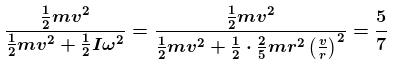*Answer can only contain numeric values
QUESTION: 2

### If the radius of the earth becomes half of its present value, with its mass remaining the same, the duration of one day will become (in hours).

Solution:

Conserving angular momentum of the earth w.r.t. its axis of rotation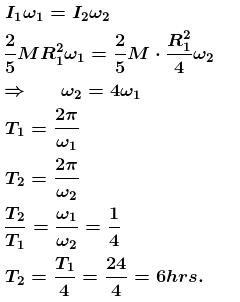*Answer can only contain numeric values
QUESTION: 3

### A uniform rod of mass m and length L is suspended with two massless strings as shown in the figure. If the rod is a rest in a horizontal position the value of tension in two strings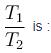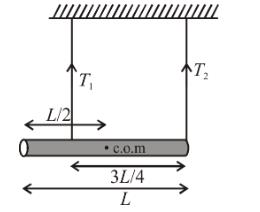Solution:

By equating force in vertical direction

T1 + T2 = mg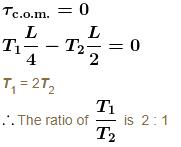*Answer can only contain numeric values
QUESTION: 4

A solid sphere and a solid cylinder having the same mass and radius, roll down the same incline. The sum of their acceleration (in ms–2) will be :

Solution: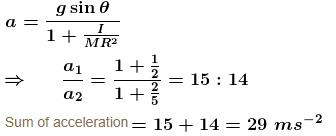*Answer can only contain numeric values
QUESTION: 5

A disc of mass M and radius R = 3m is suspended in a vertical plane by a horizontal axis passing through its centre. After sticking an object of same mass M at its rim the mass is raised to the position of maximum height. Now this unstable system is released. When the object passes the position right below the centre of angular velocity (in ms–2) of the system would be :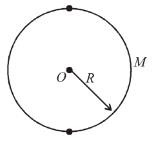Solution:

Using energy conservation P.E. = K.E.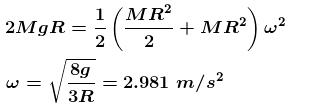*Answer can only contain numeric values
QUESTION: 6

A uniform disc m and radius R is rolling down a rough inclined plane which makes an angle 30° with the horizontal. If the coefficient of static and kinetic friction are each to µ and the only forces acting are gravitational and frictional, then the magnitude of the frictional force acting on the disc is given by mg/a. Find the value of  α?

Solution:

Under the given conditions only possibility is that friction is upwards and it accelerates downwards as shown below :

The equations of motion are :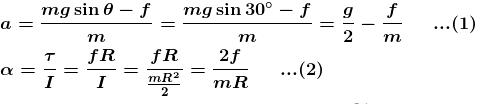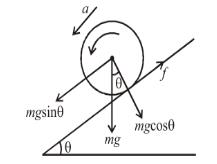For rolling (no slipping)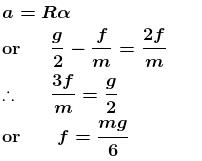*Answer can only contain numeric values
QUESTION: 7

A box of dimensions ℓ = 4m and b = 2m is kept on a truck moving with an acceleration a. If box does not slide. Maximum acceleration in ms–2 for it to remain in equilibrium (w.r.t truck) is : (Take g = 10 ms–2)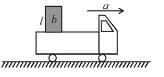Solution: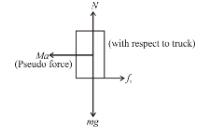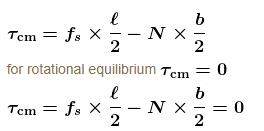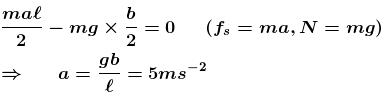*Answer can only contain numeric values
QUESTION: 8

T shaped object with dimensions shown in the figure, is lying on a smooth floor. A force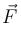is applied at the point P parallel to AB, such that the object has only the translation motion without rotation. Find the location of P with respect to C in meter, when ℓ = 3m: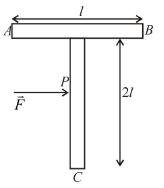Solution:

For pure translatory motion, net torque about centre of mass should be zero. Thusis applied at centre of mass of system.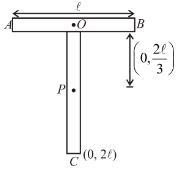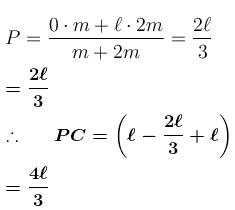PC = 4m

*Answer can only contain numeric values
QUESTION: 9

In the pulley system shown, if radii of the bigger and smaller pulley are  2m and 1m respectively and the acceleration of block A is 5 m/s2 in the downward direction, then the acceleration (in ms–2) of block will be :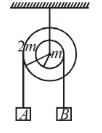Solution: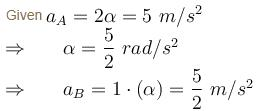*Answer can only contain numeric values
QUESTION: 10

A hole of radius R/2 is cut from a thin circular plate of radius R as shown in the figure. If the mass of the remaining plate is  M  then find moment of inertia in kgm2 of the plate about an axis through O perpendicular to plane, when  R = 6mM = 2kg?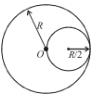Solution:

Let the mass of disc without hole =
The mass of cut hole of radius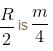∴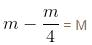or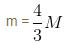Moment of inertia of given body about axis passing through

O = M of complete disc – M of cut out hole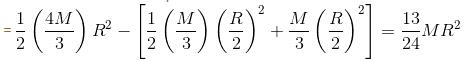On putting the value of M and R,  we get  O = 39kgm2